### Home > INT3 > Chapter Ch11 > Lesson 11.1.3 > Problem11-37

11-37.
1.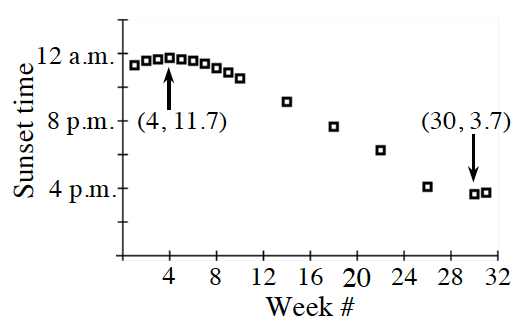Eliana is still working hard on her senior project. She has recorded the sunset time for at least one day a month through December. Her graph of the data is shown at right. Homework Help ✎

1. Write a function that models the time the sun sets as a function of the week #, where June 1 is Week 1.

2. What time should Eliana predict the sunset to take place during the first week of March (week 41)?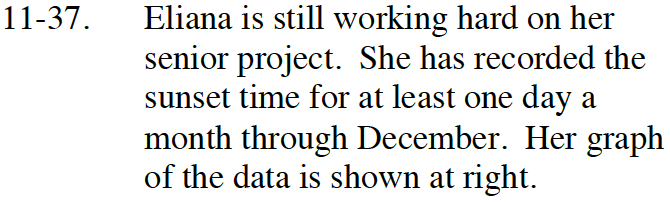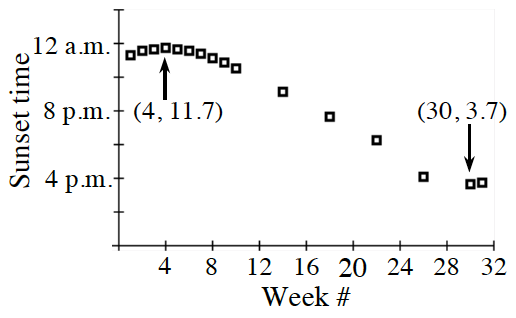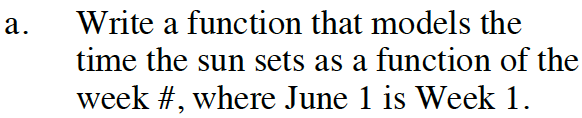One possibility is to use cosine.

Find the variables for y = acosb(xc) + d.

$\text{Amplitude is }\frac{1}{2}(\text{max time}-\text{min time}).$

$\text{Week 4 to week 30 represents }\frac{1}{2} \text{ of the period.}$

$y=4\text{cos}\left(\frac{2\pi}{52}(x-4)\right)+7.7$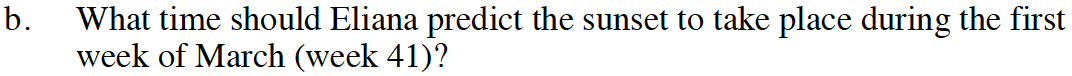Substitute x = 41 into the answer from part (a).